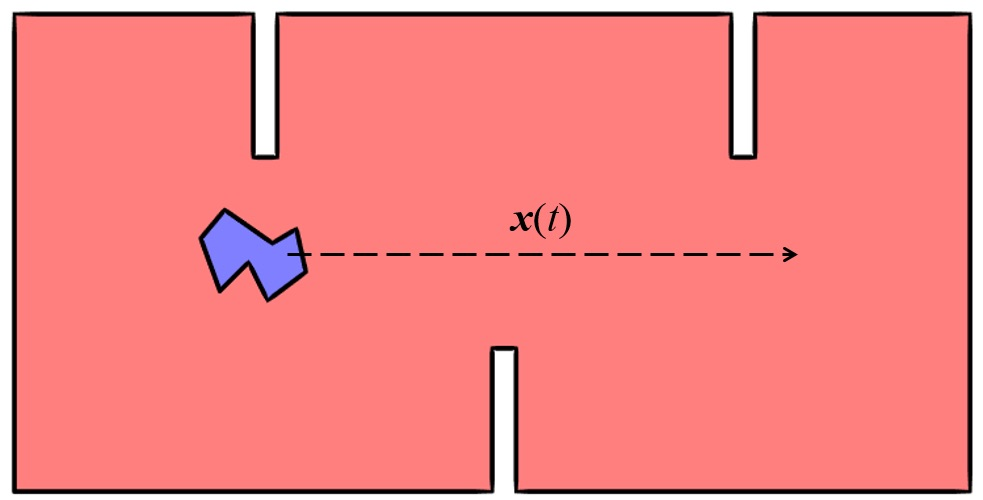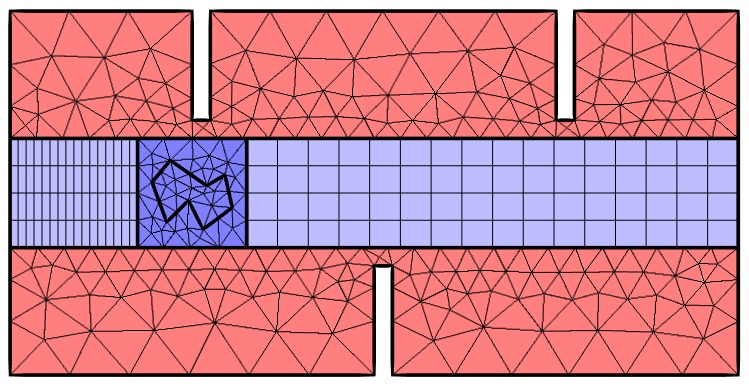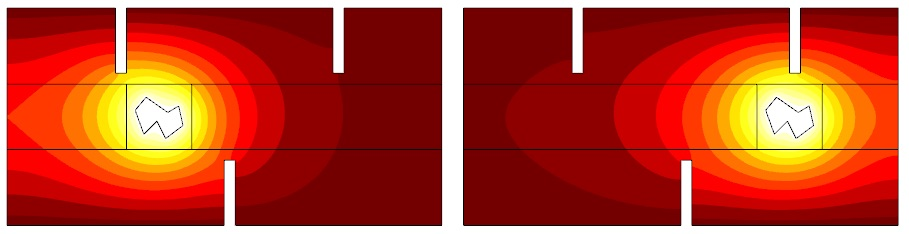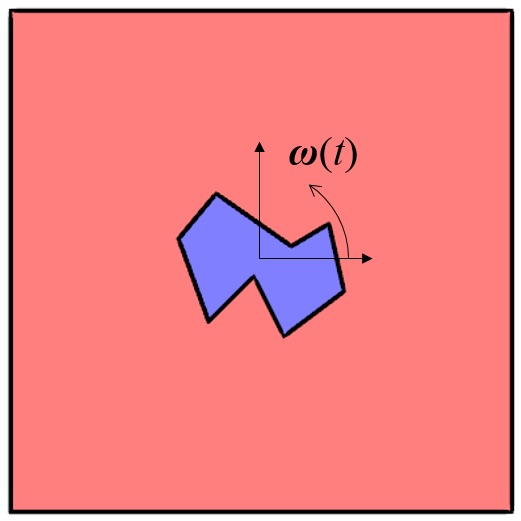变形网格接口：旋转及直线平移

直线平移通过域的对象使用滑动网格处理大型直线平移

1. 在这两个域中创建几个不同的几何对象
2. 通过形成装配体功能设定一致对
3. 通过之前设定的网格变形技巧定义直线运动
4. 使用一致对保证求解物理场的连续性保持解的连续性旋转对象\left\{ \begin{array}{c} X_r \\ Y_r\end{array} \right\} = \left[ \begin{array}{cc} cos(\omega t) & -sin (\omega t) \\ sin (\omega t) & cos (\omega t) \end{array} \right] \left\{ \begin{array}{c} X_g -X_0 \\ Y_g -Y_0 \end{array} \right\} + \left\{ \begin{array}{c} X_0 \\ Y_0 \end{array} \right\}

\begin{array}{c} d_x = X_r -X_g\\ d_y = Y_r-Y_g\end{array}

评论

1. 笑阳 雒 2019-04-02   8:19 am

您好 请三维模型中用什么物理场啊

2. Haili Wang 2019-04-15   6:55 am

三维和二维模型都是使用移动网格物理场，不过最新版本的COMSOL中不需要接口，直接在组件下定义即可，具体可以参考下述链接中的案例：http://cn.comsol.com/model/fluid-structure-interaction-361